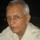Coffee Room
Discuss anything here - everything that you wish to discuss with fellow engineers.
12940 Members
Join this group to post and comment.Ammar Aziz • Oct 31, 2012

# Reactions calculation

Dear Friends, i have to face a problem in calculation of reactions from these equations
R1 + R2 + R3 = 40
0.625R1 + 0.275R2 = 9
0.275R3 - 0.35R1 = 2
0.625R3 + 0.35R2 = 16
0.175R2 + 0.45R3 - 0.175R1 = 9
Please calculate R1, R2, R3 and tell me i m very thankful to you.Ammar Aziz • Oct 31, 2012
Mention the procedure if you feel it easy!!!!There seems to be a redundancy in this. Only three independent equations are required for three variables.
There are four here.Jeffrey Arulraj • Oct 31, 2012
To much of data to solve the equations I would say there is some minor error which makes it not feasible for solving these equationsAmmar Aziz • Nov 7, 2012
bioramani
There seems to be a redundancy in this. Only three independent equations are required for three variables.
There are four here.
But sir we are here at upper position because we have more equations then no. of unknowns so what is the reason that corresponding reactions are indeterminate.Jeffrey Arulraj • Nov 7, 2012
Ammar Aziz
But sir we are here at upper position because we have more equations then no. of unknowns so what is the reason that corresponding reactions are indeterminate.
In this problem the data given has a little error and so makes solving not possible you seeAmmar Aziz • Nov 8, 2012
jeffrey samuel
In this problem the data given has a little error and so makes solving not possible you see
error of what type. i assure you that the data is correct. but if you have objection then what is the criteria on which we decide that whether the reactions are indeterminate or not.Ammar Aziz
error of what type. i assure you that the data is correct. but if you have objection then what is the criteria on which we decide that whether the reactions are indeterminate or not.
What are these reactions that you are referring to? Reaction to a force? Are these experimental equations? If these are mechanical forces and if some non linear thing like thixotropic substances are involved forces can be indeterminate.zaveri • Nov 8, 2012
is this problem from finite element methodsAmmar Aziz • Nov 12, 2012
bioramani
What are these reactions that you are referring to? Reaction to a force? Are these experimental equations? If these are mechanical forces and if some non linear thing like thixotropic substances are involved forces can be indeterminate.
actually these equations are derived from the analysis of a beam hanging horizontally with three springs. at the same time we apply two loads on two loading hangers attached with the above mentioned beam. these equations are derived by considering the equilibrium condition and applying the "sum of moments around a particular point due to all forces is zero" principle. actually the reactions which i mention in my post are the reaction forces to applied loads. and it is the springs which react to the applied load by generating upward forces in beam. now i think situation is clear.Ammar Aziz • Nov 12, 2012
it is a very simple case but i wonder what factors resist in calculation of reactionsThat part is clear now. Thanks. However, how were these four equations generated? There cannot be four independent equations in three variables.There are FIVE equations not four. Are these simultaneously applicable? Only simultaneous equations can be evaluated. If these are based on operations under different conditions, then there may be no valid solution.